2 Introducing Functions
When you type 2+2, the Wolfram Language understands it as Plus[2,2]. Plus is a function. There are more than 5000 functions built into the Wolfram Language. Arithmetic uses just a very few of these.
Compute 3+4 using the function Plus:
 In:=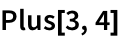Out=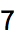Compute 1+2+3 using Plus:
 In:=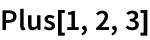Out=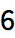The function Times does multiplication:
 In:=Out=You can put functions inside other functions:
 In:=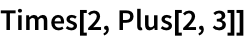Out=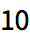The function Max finds the maximum, or largest, of a collection of numbers.
The maximum of these numbers is 7:
 In:=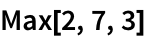Out=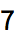The function RandomInteger picks a random integer (whole number) between 0 and whatever size you say.
Pick a random whole number between 0 and 100:
 In:=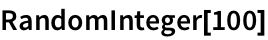Out=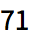Each time you ask, you get another random number:
 In:=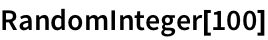Out=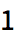2.1Compute 7+6+5 using the function Plus»
Expected output:
 Out[]=Answer & check your solution
2.2Compute 2×(3+4) using Times and Plus»
Expected output:
 Out[]=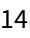Answer & check your solution
2.3Use Max to find the larger of 6×8 and 5×9. »
Expected output:
 Out[]=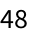Answer & check your solution
2.4Use RandomInteger to generate a random number between 0 and 1000. »
Sample expected output:
 Out[]=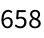Answer & check your solution
2.5Use Plus and RandomInteger to generate a number between 10 and 20. »
Sample expected output:
 Out[]=Answer & check your solution
+2.1Compute 5×4×3×2 using Times»
Expected output:
 Out[]=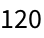Answer & check your solution
+2.2Compute 23 using Subtract»
Expected output:
 Out[]=Answer & check your solution
+2.3Compute (8+7)*(9+2) using Times and Plus»
Expected output:
 Out[]=Answer & check your solution
+2.4Compute (2689)/9 using Subtract and Divide»
Expected output:
 Out[]=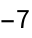Answer & check your solution
+2.5Compute 1005^2 using Subtract and Power»
Expected output:
 Out[]=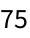Answer & check your solution
+2.6Find the larger of 3^5 and 5^3. »
Expected output:
 Out[]=Answer & check your solution
+2.7Multiply 3 and the larger of 4^3 and 3^4. »
Expected output:
 Out[]=Answer & check your solution
+2.8Add two random numbers each between 0 and 1000. »
Sample expected output:
 Out[]=Answer & check your solution
Yes. In the Wolfram Language, plus is not the same as Plus. The capital letter in Plus signifies that youre talking about the built-in (official) plus function.
Yes. Square brackets [...] are for functions; parentheses (...) are for grouping, as in 2*(3+4), not for functions.
How does one read Plus[2, 3] out loud?
Usually plus of 2 and 3; sometimes plus of 2 comma 3. [ can be read as open bracket; ] as close bracket.
Why use Plus[2, 3] instead of 2+3?
For Plus, its not necessary. But for the vast majority of functionslike Max or RandomIntegertheres no special form like +, so you have to give their names.
Can I mix Plus[...] and +?
Yes. Things like Plus[4+5, 2+3] or, for that matter, Plus[4, 5]*5 are just fine.
It means youve typed something that the Wolfram Language cant understand. See Section 47 for more information. Start by checking that your open and close brackets are matched.